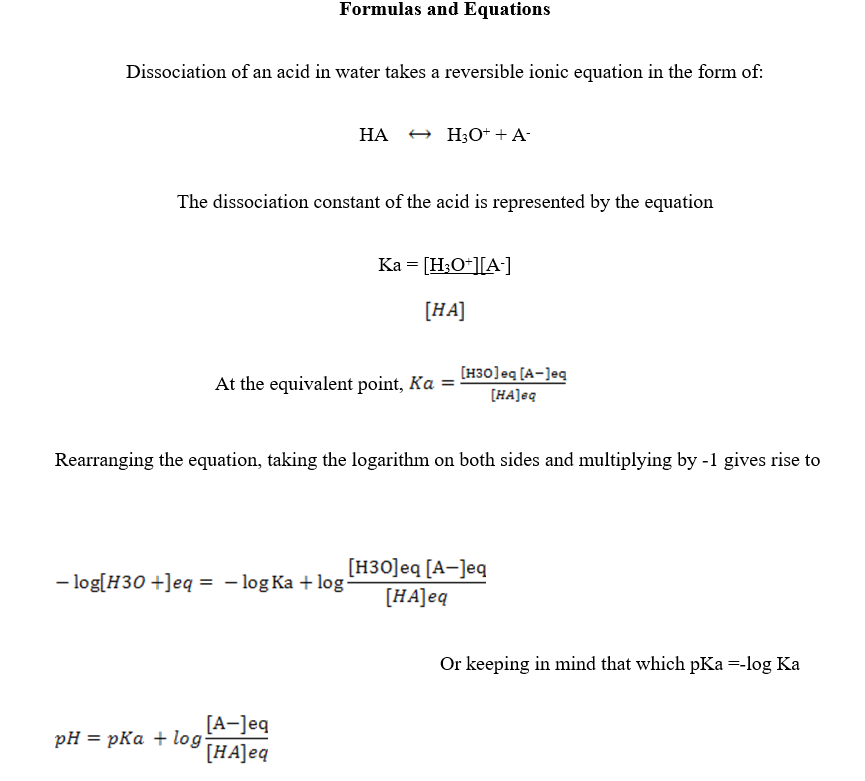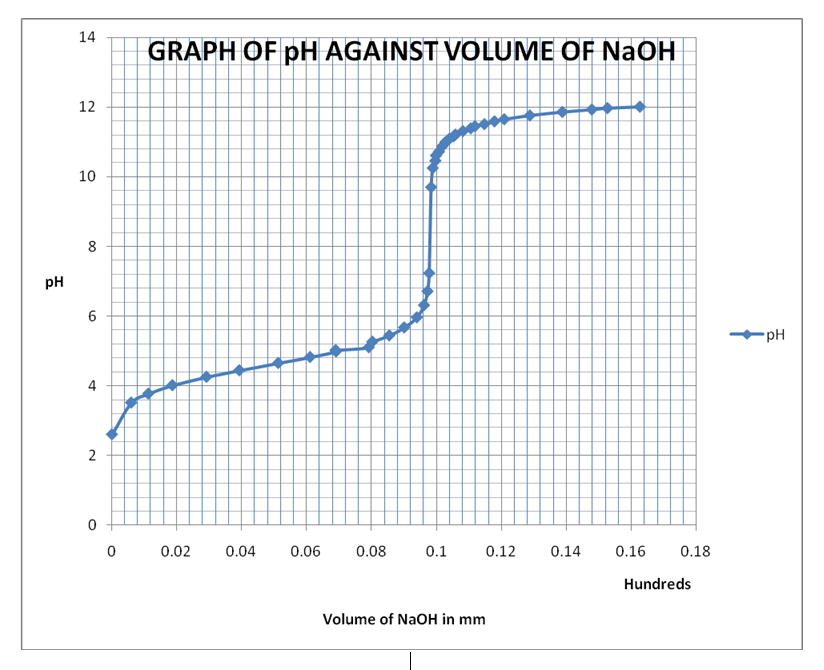All papers examples
Paper Types
Disciplines

# Determination of Ka of an Unknown Weak Acid, Lab Report Example

Pages: 4

Words: 1036

Lab Report

Theory

The objective of this experiment is to determine the Ka of unknown weak acid by following up the pH of the acid using a pH meter. The Ph meter is used to monitor the pH of the acid during an acid based titration. The obtained pairs of data comprising of pH against the volumes of the base added in millilitres in then used to determine the dissociation constant (Ka) of the weak acid.

Theoretically, weak acids react in water solutions or with bases to form hydrogen ions and halide ions of the acid. The reaction reaches the equilibrium that can be represented by the Ka of the acid. Notably, each acid has its own Ka at a given temperature of the experiment. The value of Ka is determined by use of several methods in existence. This experiment opts to use two methods to determine the Ka of the weak acid.

The first method is the use of the pH of a solution that contains a known concentration of a weak acid. In this method, a plot of pH against volume of the base added produces an S-shaped graph that is used to establish the Ka of the acid. Picking of any two points on the graph below the equivalence point with their corresponding pH helps in calculating the Ka. The second method is the use of pH at half-neutralization point in a weak acid-strong base titration. The half pH obtained is useful in the determination of the Ka of the acid as discussed in Formulas and Equations.

Formulas and EquationsThis equation is helpful in the determination of the pH of the weak acid at any given ratio of the concentration of the halide ions [A] and the concentration of the acid [HA] at the equivalence point.

When the acid is half neutralised, then [A] is equal to [HA] thus making the ratio of A and HA to be equal to 1. Since the logarithm of 1 is 0, then pH = pKa at half neutralisation.

Procedure

• A 50ml beaker was obtained and rinsed with the unknown solution of the weak acid. A 10ml pipette was also rinsed with distilled water
• 10ml of the unknown acid was pipette into a clean, dry 100ml beaker and 10ml of distilled water added
• The pH of the solution was recorded at 0 volume of the base before the start of the titration.
• Both the molarity of the base (NaOH) and the initial reading of the burette containing NaOH were recorded.
• The titration was done with the addition of larger volume of NaoH (1-2Ml) followed by a 15seconds wait and then pH recorded against the volume of NaOH used.
• The volume of base being added was reduced to 2-3 drops as the reaction approached the equivalence point.
• Increments of 2-3 ml were added after equivalence point to a pH of 12.
• Upon completion of the experiment, the pH meter was rinsed with distilled water, wiped and stored.

Data and Observations

 Initial burette reading (x) in ml Final burette reading (y) in ml Volume of NaOH used (y-x) in ml pH 25.38 25.38 0.00 2.6 25.38 25.97 0.59 3.51 25.38 26.50 1.12 3.77 25.38 27.24 1.86 4.01 25.38 28.29 2.91 4.25 25.38 29.30 3.92 4.44 25.38 30.50 5.12 4.65 25.38 31.98 6.10 4.82 25.38 32.28 6.90 4.98 25.38 32.27 6.89 5.02 25.38 32.79 7.91 5.10 25.38 33.40 8.02 5.26 25.38 33.92 8.54 5.44 25.38 34.38 9.00 5.67 25.38 34.72 9.39 5.96 25.38 34.99 9.61 6.31 25.38 35.10 9.72 6.71 25.38 35.15 9.77 7.23 25.38 35.21 9.83 9.70 25.38 35.26 9.88 10.25 25.38 35.34 9.96 10.46 25.38 35.36 9.98 10.61 25.38 35.45 10.07 10.72 25.38 35.56 10.18 10.88 25.38 35.65 10.27 10.99 25.38 35.76 10.38 11.08 25.38 35.90 10.52 11.16 25.38 35.96 10.58 11.21 25.38 36.19 10.81 11.31 25.38 36.43 11.05 11.39 25.38 36.56 11.18 11.45 25.38 36.85 11.47 11.51 25.38 37.16 11.78 11.59 25.38 37.46 12.08 11.65 25.38 38.25 12.87 11.76 25.38 39.25 13.87 11.86 25.38 40.16 14.78 11.93 25.38 40.64 15.26 11.97 25.38 41.64 16.26 12.01Calculations

From the graph, the equivalence point is at approximately pH 8.2 and at volume 10.00mm of NaOH used.

Moles of NaOH used at the equivalence volume is calculated as:

Moles = volume of NaOH used × molarity of NaOH

= 0.000997 moles.

Therefore, moles of the acid in 10ml used = 0.000997 moles.

Molarity of the acid = (1000 × 0.000997)/10

[HA] original = 0.0997M

Note: 10ml acid was diluted by adding 10ml water before titration. Therefore, the concentration of the acid used to calculate Ka is half the original concentration.

Therefore, [HA] diluted = 0.0997/2

[HA] diluted = 0.04985

Determination of Ka

Method 1: using the pH of the diluted solution

At a point on the graph of pH 4.8, volume of base = 6ml

Remember, pH = pKA + Log [A]/[HA]

4.8 = pKa + Log [A ]/[HA]

Concentration of HA

Volume of base used = 6ml. molarity = 0.0997M

Moles = 6 × 0.0997/1000= 0.0005982

pKa = 4.8 – (Log 0.0005982/0.04985)

pKa = 4.8- – 1.92

pKa = 6.72

pKa = -(log)Ka

-6.92 antilog = Ka

Ka = 12.0 ×10-6

Method 2: half-neutralisation point

pH1/2 = pKa

From the graph, pH1/2 = 4.1

Therefore, pKa = 4.1 = -(log)Ka

Ka = 10-4.1

Ka =10-6×100.9

Ka = 7.94×10-6

Discussion and Conclusion

The results indicate that monitoring the pH of a titration reaction is equally an important and accurate way of determining the dissociation constant of an acid. The experiment was successful since it enabled the calculation of the Ka of the unknown week acid HA although results from method 1 slightly differed with those from method 2. However, the accuracy of the results can be improved if both human and technical errors are minimised. Human errors result from the incorrect reading of the burette volume due to parallax effect. Technical errors arise from readings from the pH meter, which are at times inconsistent in a single data reading.

In conclusion, the Ka of the weak acid was determined successfully upon monitoring of the neutralisation reaction between a weak acid (HA) and a strong base (NaOH).Time is precious

don’t waste it!

Get instant essay
writing help!Plagiarism-free
guaranteePrivacy
guaranteeSecure
checkoutMoney back
guarantee

### The Molar Volume of Oxygen, Lab Report Example

When maintained at standard temperature and pressure, an ideal gas’s volume is the most typical illustration of the molar volume (273 K and 1.00 atm). [...]

Pages: 1

Words: 360

### Use of Gel Electrophoresis, Lab Report Example

Introduction Gel electrophoresis is used to separate DNA on the basis of its size. Due to the differential charge between the negative end of the [...]

Pages: 2

Words: 634

### Effect of Heat Stress on Beetroot and Pea Plant Cell Membranes, Lab Report Example

Introduction Both the pea plant and the beetroot are exposed to environmental changes that have the ability to alter their cellular components.  The cell membranes [...]

Pages: 8

Words: 2209

### Forensic Trace Analysis Laboratory, Lab Report Example

Circumstances of the Case The purpose of the present analysis is to examine the relationship between the actions of Ken Long, a male suspect aged [...]

Pages: 8

Words: 2107

### Thin Layer and Column Chromatography, Lab Report Example

Objective The goal of the experiment was the evaluation of organic pigments by means of the application of column chromatography in addition to a collection [...]

Pages: 4

Words: 1111

### Density and Archimedes Principle, Lab Report Example

Introduction The principle introduced by Archimedes delineates that for bodies that are proportionally or comprehensively submerged in a liquid, there is an equal and opposite [...]

Pages: 3

Words: 753

### The Molar Volume of Oxygen, Lab Report Example

When maintained at standard temperature and pressure, an ideal gas’s volume is the most typical illustration of the molar volume (273 K and 1.00 atm). [...]

Pages: 1

Words: 360

### Use of Gel Electrophoresis, Lab Report Example

Introduction Gel electrophoresis is used to separate DNA on the basis of its size. Due to the differential charge between the negative end of the [...]

Pages: 2

Words: 634

### Effect of Heat Stress on Beetroot and Pea Plant Cell Membranes, Lab Report Example

Introduction Both the pea plant and the beetroot are exposed to environmental changes that have the ability to alter their cellular components.  The cell membranes [...]

Pages: 8

Words: 2209

### Forensic Trace Analysis Laboratory, Lab Report Example

Circumstances of the Case The purpose of the present analysis is to examine the relationship between the actions of Ken Long, a male suspect aged [...]

Pages: 8

Words: 2107

### Thin Layer and Column Chromatography, Lab Report Example

Objective The goal of the experiment was the evaluation of organic pigments by means of the application of column chromatography in addition to a collection [...]

Pages: 4

Words: 1111

### Density and Archimedes Principle, Lab Report Example

Introduction The principle introduced by Archimedes delineates that for bodies that are proportionally or comprehensively submerged in a liquid, there is an equal and opposite [...]

Pages: 3

Words: 753